Courses

# Olympiad Test: Embedded Figures -2

## 10 Questions MCQ Test Science Olympiad Class 7 | Olympiad Test: Embedded Figures -2

Description
This mock test of Olympiad Test: Embedded Figures -2 for Class 7 helps you for every Class 7 entrance exam. This contains 10 Multiple Choice Questions for Class 7 Olympiad Test: Embedded Figures -2 (mcq) to study with solutions a complete question bank. The solved questions answers in this Olympiad Test: Embedded Figures -2 quiz give you a good mix of easy questions and tough questions. Class 7 students definitely take this Olympiad Test: Embedded Figures -2 exercise for a better result in the exam. You can find other Olympiad Test: Embedded Figures -2 extra questions, long questions & short questions for Class 7 on EduRev as well by searching above.
QUESTION: 1

Solution:
QUESTION: 2

Solution:
QUESTION: 3

### Directions: In each of the following questions, you are given a figure (X) followed by four alternative figures (A), (B), (C) and (D) such that figure (X) is embedded in one of them. Find out the alternative figure which contains fig. (X) as its part. Q. Find out the alternative figure which contains figure (X) as its part.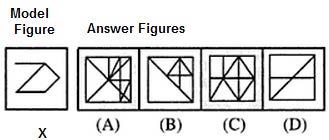Solution:
QUESTION: 4

Directions: In each of the following questions, you are given a figure (X) followed by four alternative figures (A), (B), (C) and (D) such that figure (X) is embedded in one of them. Find out the alternative figure which contains fig. (X) as its part.

Q. Find out the alternative figure which contains figure (X) as its part.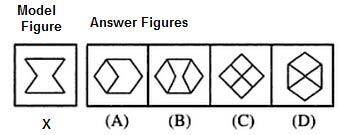Solution:
QUESTION: 5

Directions: In each of the following questions, you are given a figure (X) followed by four alternative figures (A), (B), (C) and (D) such that figure (X) is embedded in one of them. Find out the alternative figure which contains fig. (X) as its part.

Q. Find out the alternative figure which contains figure (X) as its part.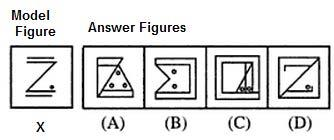Solution:
QUESTION: 6

Directions: In each of the following questions, you are given a figure (X) followed by four alternative figures (A), (B), (C) and (D) such that figure (X) is embedded in one of them. Find out the alternative figure which contains fig. (X) as its part.

Q. Find out the alternative figure which contains figure (X) as its part.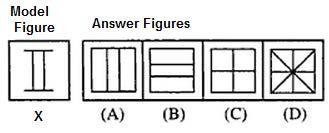Solution:
QUESTION: 7

Directions: In each of the following questions, you are given a figure (X) followed by four alternative figures (A), (B), (C) and (D) such that figure (X) is embedded in one of them. Find out the alternative figure which contains fig. (X) as its part.

Q. Find out the alternative figure which contains figure (X) as its part.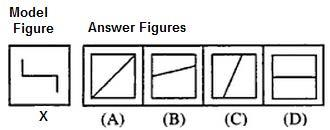Solution:
QUESTION: 8

Directions: In each of the following questions, you are given a figure (X) followed by four alternative figures (A), (B), (C) and (D) such that figure (X) is embedded in one of them. Find out the alternative figure which contains fig. (X) as its part.

Q. Find out the alternative figure which contains figure (X) as its part.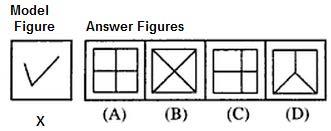Solution:
QUESTION: 9

Directions: In each of the following questions, you are given a figure (X) followed by four alternative figures (A), (B), (C) and (D) such that figure (X) is embedded in one of them. Find out the alternative figure which contains fig. (X) as its part.

Q. Find out the alternative figure which contains figure (X) as its part.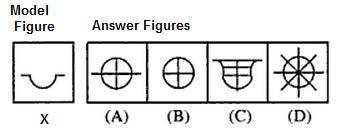Solution:
QUESTION: 10

Directions: In each of the following questions, you are given a figure (X) followed by four alternative figures (A), (B), (C) and (D) such that figure (X) is embedded in one of them. Find out the alternative figure which contains fig. (X) as its part.

Q. Find out the alternative figure which contains figure (X) as its part.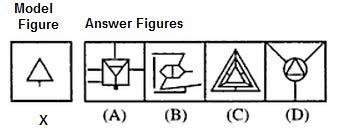Solution: